Function Repository Resource:

# RunLengthRandomnessTest

Conduct a randomness test on a sequence of random reals between 0 and 1 using run lengths of increasing subsequences

Contributed by: Emmy/Noah Blumenthal
noahb320@gmail.com
emmyb320@bu.edu
 ResourceFunction["RunLengthRandomnessTest"][sequence] uses lengths of increasing runs to test the randomness of sequence and returns an associated p-value. ResourceFunction["RunLengthRandomnessTest"][sequence,"property"] uses lengths of increasing runs and returns the associated property.

## Details and Options

Properties include:
 "TestStatistic" returns the test statistic "PValue" returns the p-value associated with the test
The test statistic is generated by creating a chi square–like statistic that measures the difference between the lengths of runs up in a sequence and the expected mean lengths of runs up in the sequence.
The test only works for sequences of random reals between 0 and 1.
ResourceFunction["RunLengthRandomnessTest"] results are valid only for sequence lengths greater than 600.
ResourceFunction["RunLengthRandomnessTest"] function performs a two-tailed test on the test statistic.

## Examples

### Basic Examples (3)

Generate a sequence of random integers:

 In:=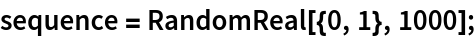Visualize the sequence:

 In:=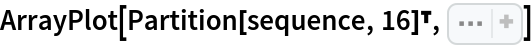Out=Apply a run length-based test:

 In:=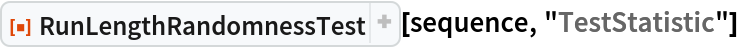Out=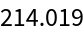In:=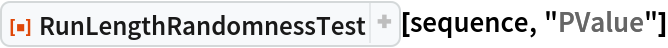Out=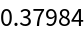### Scope (3)

Generate a sequence of random integers:

 In:=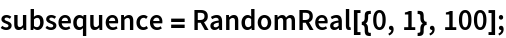In:=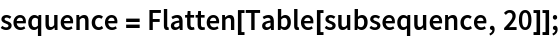Visualize the sequence:

 In:=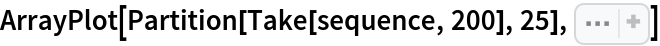Out=Attempt to reject a non-random sequence:

 In:=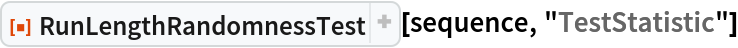Out=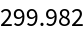In:=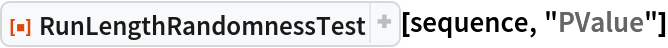Out=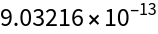### Neat Examples (1)

Visualize the sampling distribution of the test statistic:

 In:=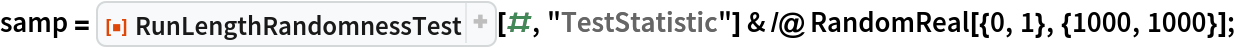In:=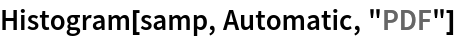Out=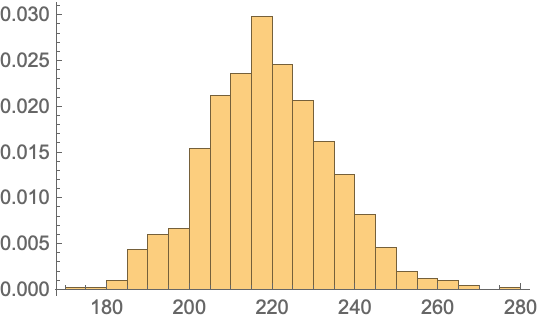In:=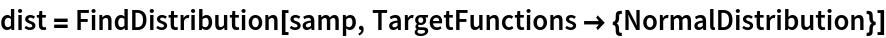Out=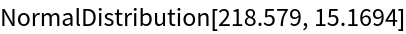In:=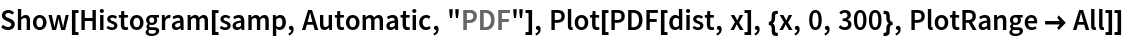Out=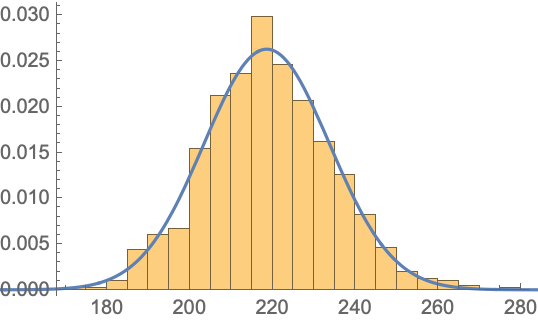Emmy Blumenthal

## Version History

• 1.0.0 – 08 July 2019